## Py Raw Ores came out

Replaces resource spawning system, so that the distances between resources are much bigger. Railway is needed then.

Moderators: Dark, orzelek

npuldon
Fast Inserter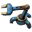Posts: 156
Joined: Thu Dec 08, 2016 8:36 am
Contact:

### Py Raw Ores came out

RSO update coming out soon?

orzelek
Smart Inserter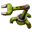Posts: 3853
Joined: Fri Apr 03, 2015 10:20 am
Contact:

### Re: Py Raw Ores came out

RSO update will come if Pyanodon or Nexela will prepare the RSO config for it.
I can help with that for syntax etc. but due to scale of Py mods I can't make a good config without a lot of guessing.
There are a lot of new ores in Py Ores mod and they might need some special treatment - from what I understand they are not like normal ores more like oil patches.

subie
Burner Inserter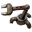Posts: 13
Joined: Tue Dec 11, 2018 7:14 am
Contact:

### Re: Py Raw Ores came out

So, I didn't want to wait anymore, and i think Py and Nexela are focusing on bug fixing for now, so I made a makeshift config file that is working.

Should be decent enough, and sets the proper starting materials, and should make the big rocks much less frequent and spread out. Only problem I can't seem to fix is that the 'liquids' still spawn 2 or 3 at a time, even if i set min and max to 1

Code: Select all

``````function fillPyRawOresConfig(config)

config["ore-aluminium"] = {
type="resource-ore",

-- general spawn params
allotment=80, -- how common resource is
spawns_per_region={min=1, max=1}, --number of chunks
richness=25000,        -- resource_ore has only one richness value - resource-liquid has min/max

size={min=20, max=30}, -- rough radius of area, too high value can produce square shaped areas
min_amount=500,

-- resource provided at starting location
-- probability: 1 = 100% chance to be in starting area
--              0 = resource is not in starting area
starting={richness=8000, size=25, probability=1}
}

config["ore-chromium"] = {
type="resource-ore",
allotment=80,
spawns_per_region={min=1, max=1},
richness=25000,
size={min=20, max=30},
min_amount=500,
starting={richness=8000, size=25, probability=0}
}

type="resource-ore",
allotment=80,
spawns_per_region={min=1, max=1},
richness=25000,
size={min=20, max=30},
min_amount=500,
starting={richness=8000, size=25, probability=1}
}

config["ore-nickel"] = {
type="resource-ore",
allotment=80,
spawns_per_region={min=1, max=1},
richness=25000,
size={min=20, max=30},
min_amount=500,
starting={richness=8000, size=25, probability=0}
}

config["ore-quartz"] = {
type="resource-ore",
allotment=80,
spawns_per_region={min=1, max=1},
richness=25000,
size={min=20, max=30},
min_amount=500,
starting={richness=8000, size=25, probability=1}
}

config["raw-coal"] = {
type="resource-ore",
allotment=80,
spawns_per_region={min=1, max=1},
richness=25000,
size={min=20, max=30},
min_amount=500,
starting={richness=8000, size=25, probability=0}
}

config["ore-tin"] = {
type="resource-ore",
allotment=80,
spawns_per_region={min=1, max=1},
richness=25000,
size={min=20, max=30},
min_amount=500,
starting={richness=8000, size=25, probability=1}
}

config["ore-titanium"] = {
type="resource-ore",
allotment=80,
spawns_per_region={min=1, max=1},
richness=25000,
size={min=20, max=30},
min_amount=500,
starting={richness=8000, size=25, probability=1}
}

config["ore-zinc"] = {
type="resource-ore",
allotment=80,
spawns_per_region={min=1, max=1},
richness=25000,
size={min=20, max=30},
min_amount=500,
starting={richness=8000, size=25, probability=0}
}

-- BIG ROCKS
config["aluminium-rock"] = {
type="resource-liquid",
minimum_amount=500000,
allotment=60,
spawns_per_region={min=1, max=1},
richness={min=500000, max=3000000}, -- richness per resource spawn
size={min=1, max=1},
useOreScaling = true
}

config["chromium-rock"] = {
type="resource-liquid",
minimum_amount=500000,
allotment=60,
spawns_per_region={min=1, max=1},
richness={min=500000, max=3000000}, -- richness per resource spawn
size={min=1, max=1},
useOreScaling = true
}

config["coal-rock"] = {
type="resource-liquid",
minimum_amount=500000,
allotment=60,
spawns_per_region={min=1, max=1},
richness={min=500000, max=3000000}, -- richness per resource spawn
size={min=1, max=1},
useOreScaling = true
}

config["copper-rock"] = {
type="resource-liquid",
minimum_amount=500000,
allotment=60,
spawns_per_region={min=1, max=1},
richness={min=500000, max=3000000}, -- richness per resource spawn
size={min=1, max=1},
useOreScaling = true
}

config["iron-rock"] = {
type="resource-liquid",
minimum_amount=500000,
allotment=60,
spawns_per_region={min=1, max=1},
richness={min=500000, max=3000000}, -- richness per resource spawn
size={min=1, max=1},
useOreScaling = true
}

type="resource-liquid",
minimum_amount=500000,
allotment=60,
spawns_per_region={min=1, max=1},
richness={min=500000, max=3000000}, -- richness per resource spawn
size={min=1, max=1},
useOreScaling = true
}

config["nexelit-rock"] = {
type="resource-liquid",
minimum_amount=500000,
allotment=60,
spawns_per_region={min=1, max=1},
richness={min=500000, max=3000000}, -- richness per resource spawn
size={min=1, max=1},
useOreScaling = true
}

config["nickel-rock"] = {
type="resource-liquid",
minimum_amount=500000,
allotment=60,
spawns_per_region={min=1, max=1},
richness={min=500000, max=3000000}, -- richness per resource spawn
size={min=1, max=1},
useOreScaling = true
}

config["phosphate-rock-02"] = {
type="resource-liquid",
minimum_amount=500000,
allotment=60,
spawns_per_region={min=1, max=1},
richness={min=500000, max=3000000}, -- richness per resource spawn
size={min=1, max=1},
useOreScaling = true
}

config["quartz-rock"] = {
type="resource-liquid",
minimum_amount=500000,
allotment=60,
spawns_per_region={min=1, max=1},
richness={min=500000, max=3000000}, -- richness per resource spawn
size={min=1, max=1},
useOreScaling = true
}

config["salt-rock"] = {
type="resource-liquid",
minimum_amount=100000,
allotment=60,
spawns_per_region={min=1, max=1},
richness={min=100000, max=1000000}, -- richness per resource spawn
size={min=1, max=3},
useOreScaling = false,

starting={richness=75000, size=1, probability=.5}
}

config["tin-rock"] = {
type="resource-liquid",
minimum_amount=500000,
allotment=60,
spawns_per_region={min=1, max=1},
richness={min=500000, max=3000000}, -- richness per resource spawn
size={min=1, max=1},
useOreScaling = true
}

config["titanium-rock"] = {
type="resource-liquid",
minimum_amount=500000,
allotment=60,
spawns_per_region={min=1, max=1},
richness={min=500000, max=3000000}, -- richness per resource spawn
size={min=1, max=1},
useOreScaling = true
}

config["uranium-rock"] = {
type="resource-liquid",
minimum_amount=500000,
allotment=60,
spawns_per_region={min=1, max=1},
richness={min=500000, max=3000000}, -- richness per resource spawn
size={min=1, max=1},
useOreScaling = true
}

config["zinc-rock"] = {
type="resource-liquid",
minimum_amount=500000,
allotment=60,
spawns_per_region={min=1, max=1},
richness={min=500000, max=3000000}, -- richness per resource spawn
size={min=1, max=1},
useOreScaling = true
}

end

``````

orzelek
Smart InserterPosts: 3853
Joined: Fri Apr 03, 2015 10:20 am
Contact:

### Re: Py Raw Ores came out

subie wrote:
Tue Dec 11, 2018 8:31 am
So, I didn't want to wait anymore, and i think Py and Nexela are focusing on bug fixing for now, so I made a makeshift config file that is working.

Should be decent enough, and sets the proper starting materials, and should make the big rocks much less frequent and spread out. Only problem I can't seem to fix is that the 'liquids' still spawn 2 or 3 at a time, even if i set min and max to 1

Code: Select all

``````function fillPyRawOresConfig(config)

config["ore-aluminium"] = {
type="resource-ore",

-- general spawn params
allotment=80, -- how common resource is
spawns_per_region={min=1, max=1}, --number of chunks
richness=25000,        -- resource_ore has only one richness value - resource-liquid has min/max

size={min=20, max=30}, -- rough radius of area, too high value can produce square shaped areas
min_amount=500,

-- resource provided at starting location
-- probability: 1 = 100% chance to be in starting area
--              0 = resource is not in starting area
starting={richness=8000, size=25, probability=1}
}

config["ore-chromium"] = {
type="resource-ore",
allotment=80,
spawns_per_region={min=1, max=1},
richness=25000,
size={min=20, max=30},
min_amount=500,
starting={richness=8000, size=25, probability=0}
}

type="resource-ore",
allotment=80,
spawns_per_region={min=1, max=1},
richness=25000,
size={min=20, max=30},
min_amount=500,
starting={richness=8000, size=25, probability=1}
}

config["ore-nickel"] = {
type="resource-ore",
allotment=80,
spawns_per_region={min=1, max=1},
richness=25000,
size={min=20, max=30},
min_amount=500,
starting={richness=8000, size=25, probability=0}
}

config["ore-quartz"] = {
type="resource-ore",
allotment=80,
spawns_per_region={min=1, max=1},
richness=25000,
size={min=20, max=30},
min_amount=500,
starting={richness=8000, size=25, probability=1}
}

config["raw-coal"] = {
type="resource-ore",
allotment=80,
spawns_per_region={min=1, max=1},
richness=25000,
size={min=20, max=30},
min_amount=500,
starting={richness=8000, size=25, probability=0}
}

config["ore-tin"] = {
type="resource-ore",
allotment=80,
spawns_per_region={min=1, max=1},
richness=25000,
size={min=20, max=30},
min_amount=500,
starting={richness=8000, size=25, probability=1}
}

config["ore-titanium"] = {
type="resource-ore",
allotment=80,
spawns_per_region={min=1, max=1},
richness=25000,
size={min=20, max=30},
min_amount=500,
starting={richness=8000, size=25, probability=1}
}

config["ore-zinc"] = {
type="resource-ore",
allotment=80,
spawns_per_region={min=1, max=1},
richness=25000,
size={min=20, max=30},
min_amount=500,
starting={richness=8000, size=25, probability=0}
}

-- BIG ROCKS
config["aluminium-rock"] = {
type="resource-liquid",
minimum_amount=500000,
allotment=60,
spawns_per_region={min=1, max=1},
richness={min=500000, max=3000000}, -- richness per resource spawn
size={min=1, max=1},
useOreScaling = true
}

config["chromium-rock"] = {
type="resource-liquid",
minimum_amount=500000,
allotment=60,
spawns_per_region={min=1, max=1},
richness={min=500000, max=3000000}, -- richness per resource spawn
size={min=1, max=1},
useOreScaling = true
}

config["coal-rock"] = {
type="resource-liquid",
minimum_amount=500000,
allotment=60,
spawns_per_region={min=1, max=1},
richness={min=500000, max=3000000}, -- richness per resource spawn
size={min=1, max=1},
useOreScaling = true
}

config["copper-rock"] = {
type="resource-liquid",
minimum_amount=500000,
allotment=60,
spawns_per_region={min=1, max=1},
richness={min=500000, max=3000000}, -- richness per resource spawn
size={min=1, max=1},
useOreScaling = true
}

config["iron-rock"] = {
type="resource-liquid",
minimum_amount=500000,
allotment=60,
spawns_per_region={min=1, max=1},
richness={min=500000, max=3000000}, -- richness per resource spawn
size={min=1, max=1},
useOreScaling = true
}

type="resource-liquid",
minimum_amount=500000,
allotment=60,
spawns_per_region={min=1, max=1},
richness={min=500000, max=3000000}, -- richness per resource spawn
size={min=1, max=1},
useOreScaling = true
}

config["nexelit-rock"] = {
type="resource-liquid",
minimum_amount=500000,
allotment=60,
spawns_per_region={min=1, max=1},
richness={min=500000, max=3000000}, -- richness per resource spawn
size={min=1, max=1},
useOreScaling = true
}

config["nickel-rock"] = {
type="resource-liquid",
minimum_amount=500000,
allotment=60,
spawns_per_region={min=1, max=1},
richness={min=500000, max=3000000}, -- richness per resource spawn
size={min=1, max=1},
useOreScaling = true
}

config["phosphate-rock-02"] = {
type="resource-liquid",
minimum_amount=500000,
allotment=60,
spawns_per_region={min=1, max=1},
richness={min=500000, max=3000000}, -- richness per resource spawn
size={min=1, max=1},
useOreScaling = true
}

config["quartz-rock"] = {
type="resource-liquid",
minimum_amount=500000,
allotment=60,
spawns_per_region={min=1, max=1},
richness={min=500000, max=3000000}, -- richness per resource spawn
size={min=1, max=1},
useOreScaling = true
}

config["salt-rock"] = {
type="resource-liquid",
minimum_amount=100000,
allotment=60,
spawns_per_region={min=1, max=1},
richness={min=100000, max=1000000}, -- richness per resource spawn
size={min=1, max=3},
useOreScaling = false,

starting={richness=75000, size=1, probability=.5}
}

config["tin-rock"] = {
type="resource-liquid",
minimum_amount=500000,
allotment=60,
spawns_per_region={min=1, max=1},
richness={min=500000, max=3000000}, -- richness per resource spawn
size={min=1, max=1},
useOreScaling = true
}

config["titanium-rock"] = {
type="resource-liquid",
minimum_amount=500000,
allotment=60,
spawns_per_region={min=1, max=1},
richness={min=500000, max=3000000}, -- richness per resource spawn
size={min=1, max=1},
useOreScaling = true
}

config["uranium-rock"] = {
type="resource-liquid",
minimum_amount=500000,
allotment=60,
spawns_per_region={min=1, max=1},
richness={min=500000, max=3000000}, -- richness per resource spawn
size={min=1, max=1},
useOreScaling = true
}

config["zinc-rock"] = {
type="resource-liquid",
minimum_amount=500000,
allotment=60,
spawns_per_region={min=1, max=1},
richness={min=500000, max=3000000}, -- richness per resource spawn
size={min=1, max=1},
useOreScaling = true
}

end

``````
Thats a quick copy/paste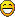Thanks for this I can add it to RSO soon-ish and post some unofficial version for playtesting.
I know why rocks don't end up solitary and it would need a code fix - it tries to scale size with distance also and rolls randomly for amount then. I'm not sure if it's that important to make them solitary?
I looked a bit at how PyOres works currently and I'm not sure if all is ok there - rocks ended up being 8M all around the place event at pretty distant locations. It looked like there is no richness scaling on them at all.

pyanodon
Smart InserterPosts: 1878
Joined: Wed Apr 20, 2016 4:42 pm
Contact:

### Re: Py Raw Ores came out

He did a great job on it!! Thank you for that. The actual value base amount of the rocks is 8mi.

Just 3 things:

1- the rocks can have their amount increased with distance, its actually a good idea, so go for it.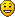2- i would put 1 in the probability of salt rock since its considered a key resource in early game, thats the only rock that people will need early and in the starting base.
3- If possible, is important the rocks being solitary. Not a "must be", but would made the thing even more fun and "realistic"
pY Coal processing mod
Discord: Pyanodon #5791

nagapito
Filter Inserter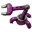Posts: 358
Joined: Fri Jul 29, 2016 12:18 am
Contact:

### Re: Py Raw Ores came out

subie wrote:
Tue Dec 11, 2018 8:31 am
So, I didn't want to wait anymore, and i think Py and Nexela are focusing on bug fixing for now, so I made a makeshift config file that is working.

Should be decent enough, and sets the proper starting materials, and should make the big rocks much less frequent and spread out. Only problem I can't seem to fix is that the 'liquids' still spawn 2 or 3 at a time, even if i set min and max to 1
Thank you so much for this!
I was going nuts with the spam of ores!

orzelek
Smart InserterPosts: 3853
Joined: Fri Apr 03, 2015 10:20 am
Contact:

### Re: Py Raw Ores came out

I've released new RSO version with the config.

chrisdec
Fast InserterPosts: 216
Joined: Fri May 27, 2016 7:50 pm
Contact:

### Re: Py Raw Ores came out

thank you orzelek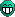Rue99
Long Handed Inserter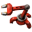Posts: 84
Joined: Wed Mar 26, 2014 7:07 am
Contact:

### Re: Py Raw Ores came out

The PyOres config seems to work very, very well. It's an opportunity for me to say thank you Orzelek, RSO is one of the key mods that has kept me playing Factorio for so many years. Kudos on a great piece of work!

orzelek
Smart InserterPosts: 3853
Joined: Fri Apr 03, 2015 10:20 am
Contact:

### Re: Py Raw Ores came out

Actual config was made by subie - I just tuned it a bit and I do think it might not be balanced in any way.
Basically all the ores and rocks are pretty much identical in spawns - I have no real data to say what should be more or less common. And reading through Py mods code is not.. pretty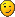Any comments on balacing would be useful.

kingarthur
Smart InserterPosts: 1400
Joined: Sun Jun 15, 2014 11:39 am
Contact:

### Re: Py Raw Ores came out

orzelek wrote:
Sat Dec 15, 2018 9:53 pm
Actual config was made by subie - I just tuned it a bit and I do think it might not be balanced in any way.
Basically all the ores and rocks are pretty much identical in spawns - I have no real data to say what should be more or less common. And reading through Py mods code is not.. prettyAny comments on balacing would be useful.
seems alright to me for the most part. only real issue is at least on default quartz seems to end up a little to rare out of five games a loaded in and scan a wide area then used resource labels to scan for everything i only found a couple of quartz patches and maybe a few rocks. one map had one non start area patch. 3 had 2 or 3 patch spread around the edge of the area revealed. slightly larger than the zoom level where labels disappear. only the one game had a decent amount(6) of quartz patches in that same space. quartz is probably the most important early game resource patch to need a spare. its the only ingredient that cant be farmed that goes into red science and the crystal mine is a huge building compared to the normal electric miner. i dont think the actual amounts in the patch are bad just real had to utilize it when you got to use a giant 5X5 or might be 6x6 building on patch that normally fit like 30 odd electric miners.

sorry for the rambling wall of word vomit.

tl:dr. if you can maybe make the quartz patches spawn over more area per patch but maybe keep the total ore amount the same.

aklesey1
Smart InserterPosts: 1841
Joined: Sun May 18, 2014 3:45 pm
Contact:

### Re: Py Raw Ores came out

subie wrote:
Tue Dec 11, 2018 8:31 am
So, I didn't want to wait anymore, and i think Py and Nexela are focusing on bug fixing for now, so I made a makeshift config file that is working.

Should be decent enough, and sets the proper starting materials, and should make the big rocks much less frequent and spread out. Only problem I can't seem to fix is that the 'liquids' still spawn 2 or 3 at a time, even if i set min and max to 1

Code: Select all

``````function fillPyRawOresConfig(config)

config["ore-aluminium"] = {
type="resource-ore",

-- general spawn params
allotment=80, -- how common resource is
spawns_per_region={min=1, max=1}, --number of chunks
richness=25000,        -- resource_ore has only one richness value - resource-liquid has min/max

size={min=20, max=30}, -- rough radius of area, too high value can produce square shaped areas
min_amount=500,

-- resource provided at starting location
-- probability: 1 = 100% chance to be in starting area
--              0 = resource is not in starting area
starting={richness=8000, size=25, probability=1}
}

config["ore-chromium"] = {
type="resource-ore",
allotment=80,
spawns_per_region={min=1, max=1},
richness=25000,
size={min=20, max=30},
min_amount=500,
starting={richness=8000, size=25, probability=0}
}

type="resource-ore",
allotment=80,
spawns_per_region={min=1, max=1},
richness=25000,
size={min=20, max=30},
min_amount=500,
starting={richness=8000, size=25, probability=1}
}

config["ore-nickel"] = {
type="resource-ore",
allotment=80,
spawns_per_region={min=1, max=1},
richness=25000,
size={min=20, max=30},
min_amount=500,
starting={richness=8000, size=25, probability=0}
}

config["ore-quartz"] = {
type="resource-ore",
allotment=80,
spawns_per_region={min=1, max=1},
richness=25000,
size={min=20, max=30},
min_amount=500,
starting={richness=8000, size=25, probability=1}
}

config["raw-coal"] = {
type="resource-ore",
allotment=80,
spawns_per_region={min=1, max=1},
richness=25000,
size={min=20, max=30},
min_amount=500,
starting={richness=8000, size=25, probability=0}
}

config["ore-tin"] = {
type="resource-ore",
allotment=80,
spawns_per_region={min=1, max=1},
richness=25000,
size={min=20, max=30},
min_amount=500,
starting={richness=8000, size=25, probability=1}
}

config["ore-titanium"] = {
type="resource-ore",
allotment=80,
spawns_per_region={min=1, max=1},
richness=25000,
size={min=20, max=30},
min_amount=500,
starting={richness=8000, size=25, probability=1}
}

config["ore-zinc"] = {
type="resource-ore",
allotment=80,
spawns_per_region={min=1, max=1},
richness=25000,
size={min=20, max=30},
min_amount=500,
starting={richness=8000, size=25, probability=0}
}

-- BIG ROCKS
config["aluminium-rock"] = {
type="resource-liquid",
minimum_amount=500000,
allotment=60,
spawns_per_region={min=1, max=1},
richness={min=500000, max=3000000}, -- richness per resource spawn
size={min=1, max=1},
useOreScaling = true
}

config["chromium-rock"] = {
type="resource-liquid",
minimum_amount=500000,
allotment=60,
spawns_per_region={min=1, max=1},
richness={min=500000, max=3000000}, -- richness per resource spawn
size={min=1, max=1},
useOreScaling = true
}

config["coal-rock"] = {
type="resource-liquid",
minimum_amount=500000,
allotment=60,
spawns_per_region={min=1, max=1},
richness={min=500000, max=3000000}, -- richness per resource spawn
size={min=1, max=1},
useOreScaling = true
}

config["copper-rock"] = {
type="resource-liquid",
minimum_amount=500000,
allotment=60,
spawns_per_region={min=1, max=1},
richness={min=500000, max=3000000}, -- richness per resource spawn
size={min=1, max=1},
useOreScaling = true
}

config["iron-rock"] = {
type="resource-liquid",
minimum_amount=500000,
allotment=60,
spawns_per_region={min=1, max=1},
richness={min=500000, max=3000000}, -- richness per resource spawn
size={min=1, max=1},
useOreScaling = true
}

type="resource-liquid",
minimum_amount=500000,
allotment=60,
spawns_per_region={min=1, max=1},
richness={min=500000, max=3000000}, -- richness per resource spawn
size={min=1, max=1},
useOreScaling = true
}

config["nexelit-rock"] = {
type="resource-liquid",
minimum_amount=500000,
allotment=60,
spawns_per_region={min=1, max=1},
richness={min=500000, max=3000000}, -- richness per resource spawn
size={min=1, max=1},
useOreScaling = true
}

config["nickel-rock"] = {
type="resource-liquid",
minimum_amount=500000,
allotment=60,
spawns_per_region={min=1, max=1},
richness={min=500000, max=3000000}, -- richness per resource spawn
size={min=1, max=1},
useOreScaling = true
}

config["phosphate-rock-02"] = {
type="resource-liquid",
minimum_amount=500000,
allotment=60,
spawns_per_region={min=1, max=1},
richness={min=500000, max=3000000}, -- richness per resource spawn
size={min=1, max=1},
useOreScaling = true
}

config["quartz-rock"] = {
type="resource-liquid",
minimum_amount=500000,
allotment=60,
spawns_per_region={min=1, max=1},
richness={min=500000, max=3000000}, -- richness per resource spawn
size={min=1, max=1},
useOreScaling = true
}

config["salt-rock"] = {
type="resource-liquid",
minimum_amount=100000,
allotment=60,
spawns_per_region={min=1, max=1},
richness={min=100000, max=1000000}, -- richness per resource spawn
size={min=1, max=3},
useOreScaling = false,

starting={richness=75000, size=1, probability=.5}
}

config["tin-rock"] = {
type="resource-liquid",
minimum_amount=500000,
allotment=60,
spawns_per_region={min=1, max=1},
richness={min=500000, max=3000000}, -- richness per resource spawn
size={min=1, max=1},
useOreScaling = true
}

config["titanium-rock"] = {
type="resource-liquid",
minimum_amount=500000,
allotment=60,
spawns_per_region={min=1, max=1},
richness={min=500000, max=3000000}, -- richness per resource spawn
size={min=1, max=1},
useOreScaling = true
}

config["uranium-rock"] = {
type="resource-liquid",
minimum_amount=500000,
allotment=60,
spawns_per_region={min=1, max=1},
richness={min=500000, max=3000000}, -- richness per resource spawn
size={min=1, max=1},
useOreScaling = true
}

config["zinc-rock"] = {
type="resource-liquid",
minimum_amount=500000,
allotment=60,
spawns_per_region={min=1, max=1},
richness={min=500000, max=3000000}, -- richness per resource spawn
size={min=1, max=1},
useOreScaling = true
}

end

``````
Yeah big thanks subie for ur and thanks to orzelek for his interesting mod, and thanks to Nexela and pyanodon for their mega-realistic overhaul mod system
But i have 1 big problem with PY Raw Ores
Its extremele hard to find "Salt Rock" - i can find all of rare resources with its rocks but i spend 1 hour to find 1 salt rock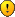- during this time, I managed to find at least 30 mountains of deposits of each of the types of resources within a radius of about 50 chunks may be more in each meter there were at least 3 types of resources, and normaly their number easily reached 5 types, I specifically decided to write down my observations in a notebook

All resource rock have parameter

Code: Select all

``useOreScaling = true``
And only salt rock have parameter

Code: Select all

``useOreScaling = false``
What influenced the decision to set this parameter in this position? When its true its more easy to find salt rocks

Do u remember how important now salt in PY RAw Ores and its usage even then PY Raw Ores not installed? causes some misunderstanding than pyanodon was not pleased with the recipe with evaporation of salt from common water without percentage chance........... I don't want to offend pyanodon but balance with salt usage but balance is skewed toward consumption
Nickname on ModPortal - Naron79

orzelek
Smart InserterPosts: 3853
Joined: Fri Apr 03, 2015 10:20 am
Contact:

### Re: Py Raw Ores came out

The useOreScaling parameter only affects how big spawns get with distance.
It's there mostly becuase fluids need a bit different scaling or you'd get stuff like thousand% pretty quickly.
It should be set on any of rocks that are of non-infinite variety.

As for having trouble to find one resource... it's possible. With PyOres you get so many of those that even with multiple spawns per region you are risking some rng misses on one of ores.
If you can provide map string I can try to generate this map and check here - if you ahve more mods then a save would be useful to sync mods and mod settings file to grab those.

aklesey1
Smart InserterPosts: 1841
Joined: Sun May 18, 2014 3:45 pm
Contact:

### Re: Py Raw Ores came out

My save was corrupted? i can't put it here i just tried to launch this save on another computer and as result i can't load anyhing

So what about the salt rocks - i started new map and set the spawn frequency to the highest
Another of my action was setting

Code: Select all

``useOreScaling = true``
it's interesting i can find many many many ancient remnants instead of salt rocks really many ancient remenats - may be i need it for PYRO aluminum chain
Nickname on ModPortal - Naron79

orzelek
Smart InserterPosts: 3853
Joined: Fri Apr 03, 2015 10:20 am
Contact:

### Re: Py Raw Ores came out

I posted a mod update that fixes the salt rocks. Starting area one was bugged and did not spawn because it was smaller then minimum amount.
As for them not being present on map - on few 2kx2k tests I had 3-5 of them spawned. It will have ore scaling now so if you find one pretty far amount should be decent.

For reference here are ore probablities per region (it should do 3 rolls per region with so many ores present):
aluminium-rock Prob: 2.4
borax Prob: 3.2
chromium-rock Prob: 2.4
coal Prob: 3.2
coal-rock Prob: 2.4
copper-ore Prob: 4.0
copper-rock Prob: 2.4
crude-oil Prob: 2.8
iron-ore Prob: 4.0
iron-rock Prob: 2.4
molybdenum-ore Prob: 2.8
nexelit-rock Prob: 2.4
nickel-rock Prob: 2.4
niobium Prob: 3.2
ore-aluminium Prob: 3.2
ore-chromium Prob: 3.2
ore-nickel Prob: 3.2
ore-quartz Prob: 3.2
ore-tin Prob: 3.2
ore-titanium Prob: 3.2
ore-zinc Prob: 3.2
phosphate-rock Prob: 2.0
phosphate-rock-02 Prob: 2.4
quartz-rock Prob: 2.4
rare-earth-bolide Prob: 2.0
raw-coal Prob: 3.2
regolites Prob: 2.4
salt-rock Prob: 2.4
stone Prob: 2.4
tin-rock Prob: 2.4
titanium-rock Prob: 2.4
uranium-ore Prob: 1.6
uranium-rock Prob: 2.4
volcanic-pipe Prob: 2.4
zinc-rock Prob: 2.4

host65
Burner InserterPosts: 14
Joined: Thu Apr 12, 2018 2:17 am
Contact:

### Re: Py Raw Ores came out

I feel that the probably of salt rocks is too low. Its a key resource compared to all the other rocks. I had to uncover 2000*2000 tiles and found 2 sources. That's too low.

I vote for doubleing the probability and for having a chance to spawn up to 3 rocks next to each other.

TwentyEighty
Fast InserterPosts: 166
Joined: Thu Jan 10, 2019 3:11 pm
Contact:

### Re: Py Raw Ores came out

Hi just wanted to echo what host65 said. One of those things is not like the others. And that's the salt rock. If you can't find an iron rock, who cares. There's a huge iron patch somewhere. But salt rock is the only way to mine salt. It needs to be a much higher probability than the other rocks. The other rocks should actually be much more rare than they are, IMO. Phosphate and salt are the only rocks that need to be common.

Phosphate actually seems to spawn ok for me, no idea why. But not nearly enough salt rocks.

TwentyEighty
Fast InserterPosts: 166
Joined: Thu Jan 10, 2019 3:11 pm
Contact:

### Re: Py Raw Ores came out

Oh, it's because phosphate rock is totally different

Code: Select all

``````	config["phosphate-rock"] = {
type="resource-liquid",
minimum_amount=40000,
allotment=50,
spawns_per_region={min=1, max=2},
richness={min=65000, max=150000}, -- richness per resource spawn
size={min=2, max=5},
useOreScaling = true,

starting={richness=75000, size=2, probability=1}
}``````

Code: Select all

``````	config["salt-rock"] = {
type="resource-liquid",
minimum_amount=100000,
allotment=60,
spawns_per_region={min=1, max=1},
richness={min=200000, max=1000000}, -- richness per resource spawn
size={min=1, max=1},
useOreScaling = true,

starting={richness=150000, size=1, probability=1}
}``````
Salt rock needs to look like phosphate rock. That would fix the problem. Salt-rock isn't a "big rock" like the others. I think the big mines are supposed to output like a blue belt or something. The salt mine is 10 salt/s IIRC.

So making the salt rock the same as phosphate would help.

orzelek
Smart InserterPosts: 3853
Joined: Fri Apr 03, 2015 10:20 am
Contact:

### Re: Py Raw Ores came out

I'm sorry but I think I need a bit more information - they are actually pretty similar in definition.

Main difference would be the size aka count of them per spawn. Is that what you mean?
Salt rock is actually much more rich but there is only one - so dropping richness by factor of lets say 4 and making them spawn in small clusters would help?

TwentyEighty
Fast InserterPosts: 166
Joined: Thu Jan 10, 2019 3:11 pm
Contact:

### Re: Py Raw Ores came out

orzelek wrote:
Tue Mar 26, 2019 11:16 pm
I'm sorry but I think I need a bit more information - they are actually pretty similar in definition.

Main difference would be the size aka count of them per spawn. Is that what you mean?
Salt rock is actually much more rich but there is only one - so dropping richness by factor of lets say 4 and making them spawn in small clusters would help?
Yes, exactly. The number of salt mines I'm able to find is more of a problem than the amount of salt in the mines, since each mine only gives 10 salt per second.